# Transformers¶

In :
from ipypublish import nb_setup


## Introduction¶

In :
#trans4
nb_setup.images_hconcat(["DL_images/trans4.png"], width=800)

Out: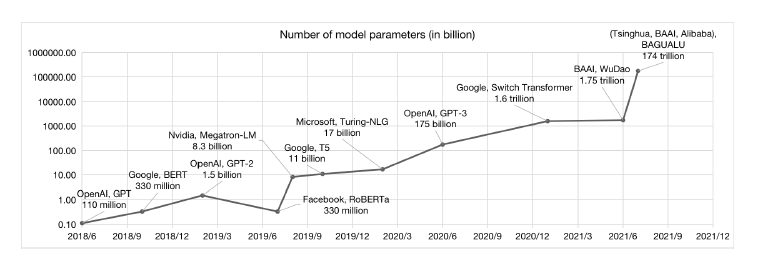Transformers are a new kind of Neural Network Architecture, that were introduced in 2017 by Vaswani et.al.. They were originally targeted at NLP applications, but since then they have been successfully applied to Image Processing as well. In NLP they overcome some of the difficulties with RNN/LSTMs, and result in much improved performance in applications such as Machine Translation. In addition, they are much better at Transfer Learning for NLP, so that Transformer models trained on huge amounts of data can be fine tuned and used for smaller datasets, just like for ConvNets.

Transformers were originally used to do Machine Translation, whereby the name comes from (they "Transform" a sentence in language 1 to language 2). Since then they have been successfully applied to other NLP applications such as Classification and Language Modeling. Indeed it was soon realized that Transformers are a versatile general purpose tool, which can be used in any Machine Learning application, as long as the input can be formatted in a way that can be ingested by them. Recently Transformers have been used for Image Processing tasks, where they have shown themselves to perform better than the best performing ConvNets.

In contrast to older architectures, Transformers have a much higher modeling capacity, which also means it is possible to scale up these models to very large sizes (see Figure trans4) with corresponding improvements in performance. Indeed some of the more recent Transformer models have hundreds of billions of parameters, which take days to train even with powerful computing infrastructure. Recall that models with large number of parameters require a correspondingly large amount of training data in order to avoid the overfitting problem. In the case of Transformers this problem was addressed by using self-supervised learning on massive text datasets.

This process, whereby larger and larger models trained on bigger and bigger datasets lead to better and better performance, has not reached its limits yet, indeed the latest Transformer features over a trillion parameters!

## Why RNNs are not Good Enough¶

In :
#rnn38
nb_setup.images_hconcat(["DL_images/rnn38.png"], width=600)

Out: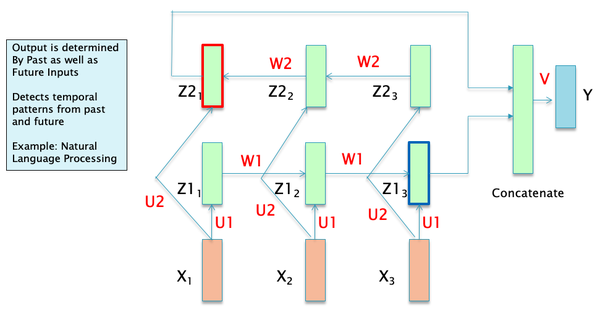Consider the Bi_directional RNN shown in Figure rnn38: Assuming that the data being fed in the network consists of NLP sequences, with the sequence $X_i, 1\le i\le N$ being the embedding or representation for the corresponding word sequence. Note that this representation does not take into account the surrounding context from the other words in the sentence. The corresponding hidden state sequence ${Z1_i}, 1\le i\le N$, can be considered to be the new representation for the sequence ${X_i}, 1\le i\le N$, such that the representation $Z1_i$ is modified by the words $X_1,...,X_i$ that came at or before $Z1_i$. In a Bi-Directional RNN, each word $X_i$ has two such representations, with the representation $Z1_i$ modified by the words $X_1,...,X_i$, and the representation $Z2_i$ modified by the words $X_{i+1},...,X_N$. Can these word representations be further improved? This RNN model has some shortfalls in this area:

• RNN representations such as $Z1_i$ or $Z2_i$ for the word $X_i$ are most influenced by other other words that are in $X_i$'s immediate neighborhood. The influence from words that are further away becomes progressively smaller due to the Vanishing Gradient problem (this is less of an issue in LSTMs, but the problem does not completely go away). It is well known that word sentences contain patterns that are strongly non-local, and this is not well captured by the RNN/LSTM type models.

• Lack of Parallelizability: Note that future RNN states cannot be computed before past RNN states have been computed. This is a significant restriction, since modern GPUs are capable of performing multiple independent computations at the same time. This causes a significant slowdown when training on very large datasets.

In order to develop better NLP models, it is important to come up with systems that are able to develop even better context dependent representations for words by allowing all the words in a sequence to interact with each other.

Just as NLP models can be improved by allowing better interactions between all the words in a sentence, as opposed to only the nearby words, it turns out that exactly the same reasoning can be applied to Image Processing as well. If we replace the word embeddings in NLP by patch embeddings in Image Processing (as we will see later in this chapter), then ConvNets can be considered to be a special type of model in which only the neighboring patches are allowed to influence each others representations. Better Image Processing models can be developed which allow ALL the patches in an image to interact with each other, which is the main idea behind a class of Transformer models for Image Processing called Vision Transformers (or ViT).

In :
#trans1
nb_setup.images_hconcat(["DL_images/trans1.png"], width=800)

Out: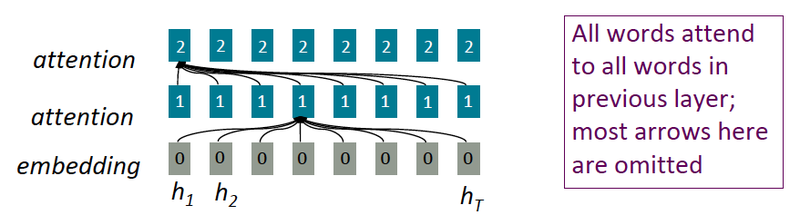## Introducing Self Attention¶

When we introduced the concept of Attention in the previous chapter (see Figure rnn65 in Chapter NLP), it was in the context of the Encoder-Decoder architecture, with Attention being used so that the words in the Encoder can influence the representation of words in the Decoder. This was referred to as Cross Attention. We are going to take this idea and modify it in a way such that the Attention mechanism can be used to allow words in the same sentence to modify each others representations, which is called Self Attention.

A possible way in which this can be done is shown in Figure trans1. The figure shows the embedded word sequence in the bottom layer followed by two layers of Self Attention. In each Self Attention layer, the representation of each of the words is modified by every other word in the sequence by using the Self Attention mechanism (an example of the connections from one of the words is shown, but the same connections exist for all the other words as well). This process can be repeated with multiple layers, with the output of layer $i$ serving as the input to layer $i+1$, as shown in the figure. The idea behind this architecture is that after several layers of Self Attention, each word develops a representation that takes into account all the other words that exist in the sentence. The exact calculations used to implement Self Attention are described in detail in the following section. Also note that the calculations for each of the words proceeds independently up the stack, which means that unlike RNNs, the words in the entire input sequence can be processed in parallel.

The idea of multilayer Self Attention as shown in the figure may remind you of the Dense Feed Forward Networks (DFN) that we encountered several chapters ago, with its dense node to node connections and multiple layers. Indeed, if we were to replace the embedding vectors with scalars (ignoring the attention calculations for a moment), then it becomes identical to the DFN architecture. From this perspective, the Multilayer Self Attention architecture can be considered to be an extention of the DFN architecture to 2D tensor or vector inputs. Hence instead of starting with a scalar sequence and transforming it with matrix multiplications in each layer (as in DFNs), we start with a vector sequence, and use the Attention mechanism to transform it into another vector sequence. This raises the interesting possibility that there should be other ways in which vector inputs can be transformed. Indeed, in addition to RNNs and LSTMs, we have already come across two ways in which this can be done:

• By using regular 2D ConvNets: We can treat the vector sequence as an 'image' and then process it by using a 2D ConvNet in the usual way

• By using 1D ConvNets: This is a more natural way to process vector sequences, and indeed in Chapter ConvNets Part 1 we saw that their performance is close to that of LSTMs when processing NLP data.

The existence of so many different ways of processing vector sequences shows that the common factor in all these designs, is a way in which the individual elements of a vector can be mixed, in both column-wise and row-wise axes, and there are multiple ways in which this can be done. What sets the Transformer apart from all these other ways of processing vectors is the fact that they have a much higher model capacity with the ability to scale up to models with hundreds of billions of parameters. This allows them to capture and model much more complex patterns. The amount of complexity in language data or image data is such that LSTM or ConvNet models are not able to capture all the interconnected patterns that exist in them and thus are capacity limited.

## Transformer Architecture¶

A Transformer consists of a set of identical modules that are stacked in a serial fashion. Note that each module has its own set of parameters. The block level structure of each module, as shown in Figure rnn87(a), consists of a Self-Attention layer followed by a Feed Forward layer.

In :
#rnn87
nb_setup.images_hconcat(["DL_images/rnn87.png"], width=800)

Out: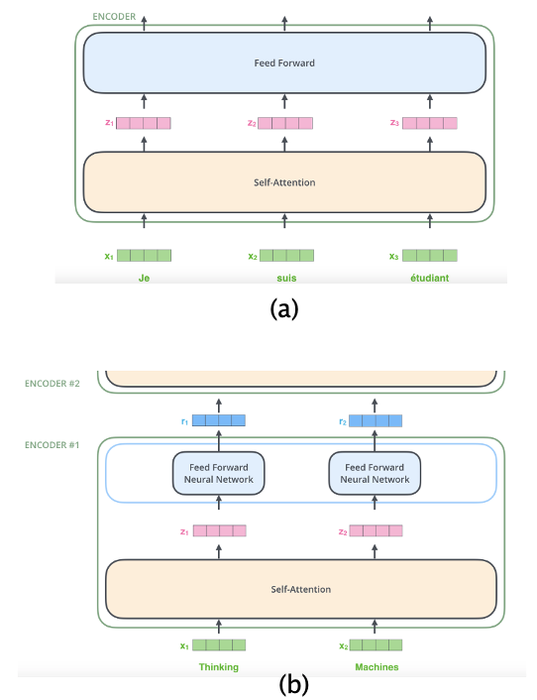Part (b) of Figure rnn87 show the progress of an input sequence (of vectors) as it traverses a module. The input $(x_1,x_2)$ is first processed by the Self Attention layer and results in the sequence $(z_1,z_2)$. This is further processed by the Dense Feed Forward Layer and the sequence $(r_1,r_2)$ is the final output for this layer. Note that each member of the sequence is propagated separately through the Self Attention and Dense layers and weight parameters are shared across all of them (each Encoder Layer has its own set of parameters though). The calculations for $x_1$ as it goes up the stack are independent from those for $x_2$, so both can proceed in parallel.

Lets first examine the Self Attention layer in greater detail:

In :
#trans2
nb_setup.images_hconcat(["DL_images/trans2.png"], width=800)

Out: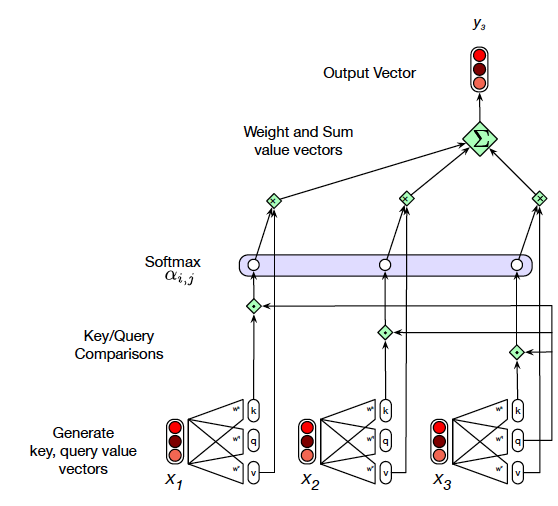In Figure trans2 we show how to go from the input $X_3$ to the output $Z_3$ of the Self Attention layer. Note that $Z_3$ is a measure of the Self Attention that $X_3$ pays to the other vectors $(X_1, X_2)$ in the input sequence. The simplest way to compute the Self Attention between two vectors is by taking their dot product, and this was the technique used for RNN based Cross Attention in the prior chapter. If we carry out this procedure, then the Self Attention between vectors $X_i$ and $X_j$ is given by $A_{ij} = X_i\cdot X_j$. These numbers can then be converted into weights $$w_{ij} = {{e^{A_{ij}}}\over{\sum_j{e^{A_{ij}}}}},\ \ i,j = 1,2,...,N$$ The output vector $Z_{i}$ is computed as a weighted sum of the input vectors $X_i$ $$Z_{i} = \sum_j w_{ij} X_j$$ This procedure represents the core of Self Attention based approach and it worked well for the RNN Cross Attention design, but note that it has the following drawback: There are no learnable parameters in these computations. We would give the Neural Network more flexibility (and thus greater capacity) if we were to change the procedure in a way that allows the network to modify the weights $w_{ij}$ during the course of the training process. In order to so, we define a new Self Attention procedure, fundamental to which are the following three vector sequences:

• Queries $(Q_1,Q_2,...,Q_N)$: The Query $Q_i$ for the $i^{th}$ input, represents the focus of Attention when this input is being processed, and is used to compare the $i^{th}$ input to all the other inputs.
• Keys $(K_1,K_2,...,K_N)$: The Key $K_j$ for the $j^{th}$ input is used to compare this input with the current focus of Attention.
• Values $(V_1,V_2,...,V_N)$: Instead of applying Self Attention to the input $(X_1,X_2,...,X_N)$ directly, it is first converted into another sequence $(V_1,V_2,...,V_N)$, called the Value Sequence. This sequence is used to compute the output for the current focus of attention.

The three sequences are derived from the input sequence $(X_1,...,X_N)$ by means of linear transformations with learnable weights.

• The Queries $(Q_1,Q_2,...,Q_N)$ are generated from the Encoder inputs by multiplication of the inputs $(X_1,X_2,...,X_N)$ with a Query matrix $W^Q$.

• The Keys $(K_1,K_2,...,K_N)$ are generated by multiplying the input $(X_1,X_2,...,X_N)$ by the Key Matrix $W^K$.

• The Values $(V_1,V_2,...,V_N)$ are generated by multiplying the input $(X_1,X_2,...,X_N)$ by the Value Matrix $W^V$.

Note that these transformations are equivalent to taking each of the vectors $X_i, i = 1,...,N$ and passing them through three separate Dense Feed Forward Networks $W^Q, W^K$ and $W^V$, as shown in Figure trans2.

Each of the vectors $X_i$ is of dimension $1\times d$, while $W^Q, W^K$ and $W^V$ are of dimensions $d\times d$. The contents of these matrices are parameters that are estimated using Gradient Descent during the training process. Once we have computed the Queries, Keys and Values, the Self Attention computation for the $i^{th}$ input $X_i$ proceeds as follows:

1. Compute a scalar valued Score $s_{ij},\ j=1,2,...,N$ associated with the $i^{th}$ and $j^{th}$ inputs by taking the inner product $$s_{ij} = Q_i^T K_j,\ \ j=1,2,...,N$$ The Score value is a measure of the similarity between these two vectors.

2. Normalize the Score values by dividing by $\sqrt{d}$ to create the sequence $s'_{ij}, j=1,2,...,N$. $$s'_{ij} = {{Q_i^T K_j}\over{\sqrt d}},\ \ j=1,2,...,N$$ This can be considered to be a type of Normalization in order to keep the results of the dot product between the Query and Key vector under control. Without this, there is danger that the dot product may become very large (or very small), which in combination with the exponentiation in the softmax (the following step) leads to numerical issues and problems in gradient propagation.

3. The normalized Scores are used to generate scalar weights $w_{ij}$ by using the Softmax function $$w_{ij} = {{e^{s'_{ij}}}\over{\sum_j{e^{s'_{ij}}}}},\ \ j=1,2,...,N$$

4. The Self Attention output vector $Z_{i}$ for the $i^{th}$ input is computed as a weighted sum of the Value vectors $$Z_{i} = \sum_j w_{ij} V_j$$

Since each of the outputs $Z_i$ can be computed independently, these calculations can be parallelized by using matrix multiplication, as follows: The vector sequence $(X_1,...,X_N)$ is packed into a matrix $X\in R^{N\times d}$, such that the $i^{th}$ row of $X$ represents the vector $X_i$. We then multiply $X$ by the matrices $W^Q, W^K$ and $W^V$, each of which are of dimension $d\times d$, to produce matrices $Q, K, V$ of dimensions $N\times d$: $$Q = XW^Q, \ \ K = XW^K, \ \ V = XW^V$$ These three matrices contain all of the Query, Key and Value vectors. By using them, the calculations in steps 1 to 4 can be reduced to a single step: $$Z = softmax({QK^T\over{\sqrt{d}}}) V$$ Note that the output vector $Z_i$ is the $i^{th}$ row of this matrix.

In :
#trans3
nb_setup.images_hconcat(["DL_images/trans3.png"], width=600)

Out: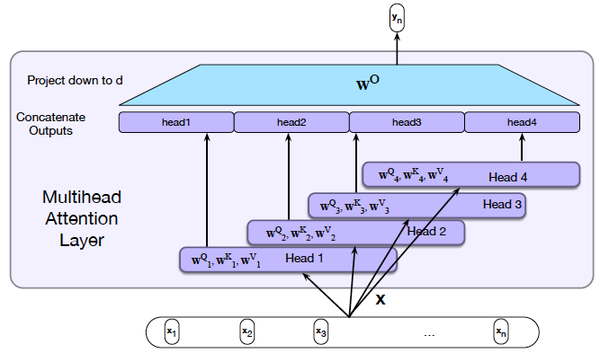The Attention weight $w_{ij}$ for the $i^{th}$ input $X_i$ is a measure of how important the $j^{th}$ input $X_j$ is in the calculation of the Self-Attention $Z_i$, which is the new representation for $X_i$. Note that this captures only one set of dependencies between the $i^{th}$ input and all the other inputs. Just as an image has multiple patterns whose capture requires multiple ConvNet filters, the Transformer model uses multiple Attention weights (and thus multiple Self-Attention values) in order to capture other dependencies between the $i^{th}$ and the other inputs.

As shown in Figure trans3, Multiple Attention Heads are implemented with the help of $H$ versions of the Query, Key and Value matrices: $(W^Q_1,...,W^Q_H),\ (W^K_1,...,W^K_H)$ and $(W^V_1,...,W^V_H)$, each of which are of dimension $N\times{d\over H}$. These are then used to compute $H$ Self Attention matrices, given by $(Z^1,...,Z^H)$, using the same computations as before, each of which are of dimension $N\times{d\over H}$. In order to generate a single output value, these $H$ matrices are first concatenated together to create a $N\times d$ matrix $\zeta = Z^1 || Z^2||... || Z^H$, followed by multiplication with another matrix $W^O$ in order to compute the final output $Z$: $$Z = \zeta W^O$$ If the matrix $W^O$ is chosen to be of dimension $d\times d$, then this results in a final Attention vector of the same size as when only one Attention Head was being used. This also means that there is no increase in either the number of parameters or amount computation in implementing additional Heads. In the original Attention paper $d = 768$ and $H = 10$.

Note: Some Self Attention implementations, such as the one that is in Keras, do not do this truncation of the individual Attention Head matrices, instead choosing to stick to the original dimensions for each of the Attention Heads. This results in a $\zeta$ matrix of size $N\times dH$, which is then multiplied by a $W^O$ matrix of size $dh\times d$ to produce the $Z$ matrix of the right size, $N\times d$.

### A Complete Transformer Block¶

In :
#TransformerBlock
nb_setup.images_hconcat(["DL_images/rnn101.png"], width=400)

Out: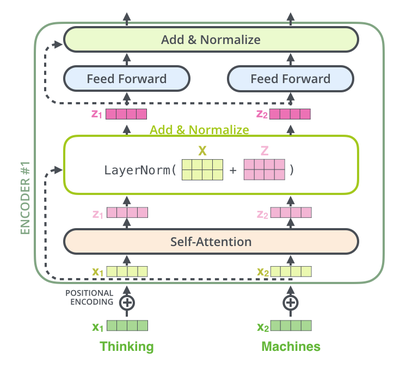Figure TransformerBlock shows a complete Encoder Layer. In addition to the Self-Attention Layer, it includes the following:

Residual Connection + Layer Normalization:

As shown in the figure, each Encoder block has two Residual Connections, one around the Self Attention Layer, and the other around the Dense Feed Forward Layer. As in ResNets (see Chapter ConvNetsPart2),each of these Residual Connections does a bypass from the input to the output of these two layers, and the vectors at either end are added together. In addition to facilitating gradient flow during backprop, these connections also create four separate paths through the Encoder Layer in the forward direction. These two properties together enable very deep networks that are nevertheless trainable, and create an Ensemble like effect when making decisions.

Each of the two Residual Connections is followed by Layer Normalization. We discussed Batch Normalization in Chapter GradientDescentTechniques in which normalization is done one feature at a time, across a batch. Layer Normalization on the other hand, carries out Normalization across features in a single training sample, as opposed to a batch. As shown below, normalization is done by computing the mean and standard deviation for the elements in a single vector. \begin{eqnarray} \mu_L & = & \frac{1}{d}\sum_{m=1}^{d}a(m) \\ \sigma_L^2 & = & \frac{1}{d}\sum_{m=1}^d (a(m)-\mu_L)^2 \\ \hat{a}(m) & = & \frac{a(m)-\mu_L}{\sqrt{\sigma_L^2+\epsilon}} \\ c(m) & = & \gamma\hat{a}(m) + \beta \end{eqnarray}

Layer Normalization was introduced by Ba, Kiros,Hinton and works better in Transformers than Batch Normalization.

Dense Feed Forward Layer:

The output of the first Layer Normalization is fed into a Dense Feed Forward Layer. The computation carried out by this layer is as follows: $$R_i = ReLU(Z_iW_1 +b_1)W_2 + b_2,\ \ i =1,...,N$$ Hence each of the vectors $Z_1,...,Z_N$ is processed independently by two DFN layers, with ReLU being applied only after the first layer. Note that all of DFNs in a layer share the same parameters, however the DFN parameters differ across layers. In the original paper, the $W_1$ matrix was of dimension $d\times 4d$, while the $W_2$ matrix was of dimension $4d\times d$. Hence the output of the DFN layer is set of vectors $R_1,...,R_N$ each of which are of dimension $1\times d$.

The output of this layer is subjected to another round of Residual connection + Layer Normalization before generating the final output of the Encoder Layer $R_i, i=1,2,...,N$. The addition of the DFN layer accomplishes the following:

• It introduces a non-linearity into the model. This is important since the Self Attention layer does not have any non-linearities.

• It serves as a mechanism for the mixing within a 'channel' and also introduces an 'inverted bottleneck' into the architecture. This is further explained in a following section.

The set of vectors $(R_1,R_2,...,R_N)$ are then passed through another Self Attention + Dense layer, as shown in Figure trans23, and this process is repeated $P$ times to finally generate the output of the Encoder Block.

The computations in a single Encoder Block can be summarized as: $$Z = LayerNorm(X + SelfAttn(X))$$ $$R = LayerNorm(Z + DFN(Z))$$

In :
#trans23
nb_setup.images_hconcat(["DL_images/trans23.png"], width=400)

Out: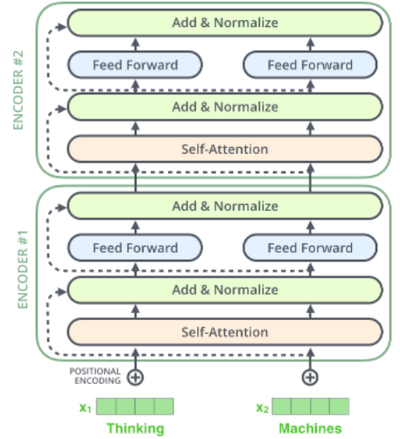## Keras Model for a Transformer Encoder¶

The following Transformer model is used to classify movie reviews from the IMDB dataset (which has already been downloaded). We start by invoking the text_dataset_from_directory function to create the training, validation and test samples.

In :
import os, pathlib, shutil, random
from tensorflow import keras
batch_size = 32
base_dir = '/Users/subirvarma/handson-ml/datasets/aclImdb'

train_ds = keras.utils.text_dataset_from_directory(
"/Users/subirvarma/handson-ml/datasets/aclImdb/train", batch_size=batch_size
)
val_ds = keras.utils.text_dataset_from_directory(
"/Users/subirvarma/handson-ml/datasets/aclImdb/val", batch_size=batch_size
)
test_ds = keras.utils.text_dataset_from_directory(
"/Users/subirvarma/handson-ml/datasets/aclImdb/test", batch_size=batch_size
)
text_only_train_ds = train_ds.map(lambda x, y: x)

Found 20000 files belonging to 2 classes.
Found 5000 files belonging to 2 classes.
Found 25000 files belonging to 2 classes.


The TextVectorization is invoked to convert the text into integers, for each sample review. Each review is restricted to 600 characters or less, and the vocabulary used is restricted to the 20,000 most frequently ocurring words found in the reviews.

In :
from tensorflow.keras import layers

max_length = 600
max_tokens = 20000
text_vectorization = layers.TextVectorization(
max_tokens=max_tokens,
output_mode="int",
output_sequence_length=max_length,
)

int_train_ds = train_ds.map(lambda x, y: (text_vectorization(x), y))
int_val_ds = val_ds.map(lambda x, y: (text_vectorization(x), y))
int_test_ds = test_ds.map(lambda x, y: (text_vectorization(x), y))


The TransformerEncoder class defines the Self Attention and Dense Feed Forward blocks in the Transformer Encoder. The Keras MultiHeadAttention function implements the Attention calculations. Its main parameters are:

• embed_dim: The size of the input vectors after embedding
• dense_dim: The number of nodes in the first DFN layer. Note that the number of nodes in the second DFN layer is equal to the embed_dim.
• key_dim: Size of Attention Heads for Query and Key
• value_dim: Size of Attention Heads for Value, defaults to embed_dim

Note that the length of the Transformer is not explicitly specified, since it is equal to the Sequence Length of the input (set to max_length=600 in the previous code block).

When the Attention function is invoked, its call arguments include:

• query: The Query Tensor of shape (B, N, d), where B is the Batch Size, N is the Sequence Length and d is the embeddding dimension size
• value: the Value Tensor, also of shape (B, N, d)
• key: Optional, if not given, then value is used for both key and value
• attention_mask: A boolean of shape (B, N, d) that prevents attention to certain positions. This is not used in the current example, but we will see it in action when we discuss Language Models using Transformers
In :
import tensorflow as tf
from tensorflow import keras
from tensorflow.keras import layers

class TransformerEncoder(layers.Layer):
def __init__(self, embed_dim, dense_dim, num_heads, **kwargs):
super().__init__(**kwargs)
self.embed_dim = embed_dim
self.dense_dim = dense_dim
# Define the Multi Headed Attention block
# Define the DFN block
self.dense_proj = keras.Sequential(
[layers.Dense(dense_dim, activation="relu"),
layers.Dense(embed_dim),]
)
self.layernorm_1 = layers.LayerNormalization()
self.layernorm_2 = layers.LayerNormalization()

attention_output = self.attention(
# Implement Residual Connection and Layer Normalization after the Self Attention block
proj_input = self.layernorm_1(inputs + attention_output)
# Send the resulting ouput through the DFN block
proj_output = self.dense_proj(proj_input)
# This is then sent through another round of Residual Connection followed by Layer Normalization
return self.layernorm_2(proj_input + proj_output)

def get_config(self):
config = super().get_config()
config.update({
"embed_dim": self.embed_dim,
"dense_dim": self.dense_dim,
})
return config

In :
vocab_size = 20000
embed_dim = 32
dense_dim = 32

inputs = keras.Input(shape=(None,), dtype="int64")
# The Embedding layer coverts the input one-hot vector of size vocab_size into an embedded vector
# of size embed_dim
x = layers.Embedding(vocab_size, embed_dim)(inputs)
# The embedded vectors are sent through the Transformer Encoder
# The output from the Encoder is sent through the GlobalMaxPooling1D layer
# which creates a single vector of size 1 X d by taking the max across the N elements
# in each row of the N X d output matrix from the Encoder
x = layers.GlobalMaxPooling1D()(x)
x = layers.Dropout(0.5)(x)
outputs = layers.Dense(1, activation="sigmoid")(x)
model = keras.Model(inputs, outputs)
model.compile(optimizer="rmsprop",
loss="binary_crossentropy",
metrics=["accuracy"])
model.summary()

Model: "model_6"
_________________________________________________________________
Layer (type)                 Output Shape              Param #
=================================================================
input_7 (InputLayer)         [(None, None)]            0
_________________________________________________________________
embedding_7 (Embedding)      (None, None, 32)          640000
_________________________________________________________________
transformer_encoder_3 (Trans (None, None, 32)          10656
_________________________________________________________________
global_max_pooling1d_3 (Glob (None, 32)                0
_________________________________________________________________
dropout_60 (Dropout)         (None, 32)                0
_________________________________________________________________
dense_71 (Dense)             (None, 1)                 33
=================================================================
Total params: 650,689
Trainable params: 650,689
Non-trainable params: 0
_________________________________________________________________


Recall that we used a LSTM based model to do IMDB classification in Chapter NLP. It is interesting to compare the number of parameters in the Transformer vs the LSTM model, and it turns out that they are approximately the same, around 650K (this assumes an LSTM with the same number of cell nodes as the size of the embed_dim in the Transformer, which is 32 in this case). More specifically the number of parameters in the LSTM part of the model and the Transformer Encoder are also about the same.

In :
model.fit(int_train_ds, validation_data=int_val_ds, epochs=15)

Epoch 1/15
625/625 [==============================] - 365s 582ms/step - loss: 0.5129 - accuracy: 0.7516 - val_loss: 0.3223 - val_accuracy: 0.8598
Epoch 2/15
625/625 [==============================] - 395s 632ms/step - loss: 0.3275 - accuracy: 0.8648 - val_loss: 0.2922 - val_accuracy: 0.8762
Epoch 3/15
625/625 [==============================] - 384s 614ms/step - loss: 0.2587 - accuracy: 0.8970 - val_loss: 0.2582 - val_accuracy: 0.8946
Epoch 4/15
625/625 [==============================] - 374s 598ms/step - loss: 0.2170 - accuracy: 0.9158 - val_loss: 0.2608 - val_accuracy: 0.8914
Epoch 5/15
625/625 [==============================] - 372s 595ms/step - loss: 0.1936 - accuracy: 0.9258 - val_loss: 0.2500 - val_accuracy: 0.9016
Epoch 6/15
625/625 [==============================] - 369s 591ms/step - loss: 0.1690 - accuracy: 0.9365 - val_loss: 0.2785 - val_accuracy: 0.8910
Epoch 7/15
625/625 [==============================] - 372s 595ms/step - loss: 0.1523 - accuracy: 0.9438 - val_loss: 0.2673 - val_accuracy: 0.8984
Epoch 8/15
625/625 [==============================] - 388s 621ms/step - loss: 0.1390 - accuracy: 0.9495 - val_loss: 0.3196 - val_accuracy: 0.8888
Epoch 9/15
625/625 [==============================] - 390s 624ms/step - loss: 0.1244 - accuracy: 0.9560 - val_loss: 0.3164 - val_accuracy: 0.8920
Epoch 10/15
625/625 [==============================] - 390s 624ms/step - loss: 0.1162 - accuracy: 0.9584 - val_loss: 0.3457 - val_accuracy: 0.8856
Epoch 11/15
625/625 [==============================] - 397s 635ms/step - loss: 0.1061 - accuracy: 0.9642 - val_loss: 0.3486 - val_accuracy: 0.8874
Epoch 12/15
625/625 [==============================] - 3833s 6s/step - loss: 0.1002 - accuracy: 0.9654 - val_loss: 0.4310 - val_accuracy: 0.8646
Epoch 13/15
625/625 [==============================] - 370s 592ms/step - loss: 0.0909 - accuracy: 0.9678 - val_loss: 0.3508 - val_accuracy: 0.8880
Epoch 14/15
625/625 [==============================] - 374s 599ms/step - loss: 0.0831 - accuracy: 0.9737 - val_loss: 0.3414 - val_accuracy: 0.8866
Epoch 15/15
625/625 [==============================] - 371s 594ms/step - loss: 0.0778 - accuracy: 0.9743 - val_loss: 0.4321 - val_accuracy: 0.8770

Out:
<keras.callbacks.History at 0x14de4f7b8>

The model.summary computed the total number of parameters in the Encoder Block to be 10,656. The spreadsheet in Figure trans5 shows how this number was arrived at.

In :
#trans5
nb_setup.images_hconcat(["DL_images/trans5.png"], width=800)

Out: